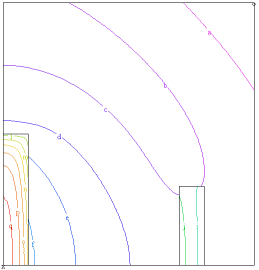﻿ Sample Problems > Applications > Heatflow > radiation_flow

Navigation:  Sample Problems > Applications > Heatflow >{ RADIATION_FLOW.PDE  This problem demonstrates the use of FlexPDE  in the solution of problems in radiative transfer.  Briefly summarized, we solve a Poisson equation  for the radiation energy density, assuming that  at every point in the domain the local  temperature has come into equilibrium with the  impinging radiation field.  We further assume that the spectral character-  istics of the radiation field are adequately  described by three average cross-sections:  the emission average, or "Planck Mean", sigmap;  the absorption average, sigmaa; and the transport  average, or "Rosseland Mean-Free-Path", lambda.  These averages may, of course, differ in various  regions, but they must be estimated by facilities  outside the scope of FlexPDE.    And finally, we assume that the radiation field  is sufficiently isotropic that Fick's Law, that  the flux is proportional to the gradient of the  energy density, is valid.  The problems shows a hot slab radiating across an  air gap and heating a distant dense slab. }variables

definitions

source         { declare the parameters, values will follow }

lambdar         { Rosseland Mean Free Path }

sigmap         { Planck Mean Emission cross-section }

sigmaa         { absorption average cross-section }

beta = 1/3     { Fick's Law proportionality factor }

materials

'air'        : source=0    sigmap=2    sigmaa=1    lambdar=10

'hot slab'   : source=100  sigmap=10   sigmaa=10   lambdar=1

'dense slab' : source=0    sigmap=10   sigmaa=10   lambdar=1

equations   { The radiation flow equation: }

boundaries

region 1   { the bounding region is tenuous }

use material 'air'

start(0,0)

natural(erad)=0       { along the bottom, a zero-flux symmetry plane }

line to (1,0)

line to (1,1) to (0,1)

natural(erad)=0       { Symmetry plane on left }

line to close

region 2   { this region has a source and large cross-section }

use material 'hot slab'

start(0,0)

line to (0.1,0) to (0.1,0.5) to (0,0.5) to close

region 3   { this opaque region is driven by radiation }

use material 'dense slab'

start(0.7,0)

line to (0.8,0) to (0.8,0.3) to (0.7,0.3) to close

monitors

plots

{ the temperature can be calculated from the assumption of equilibrium: }